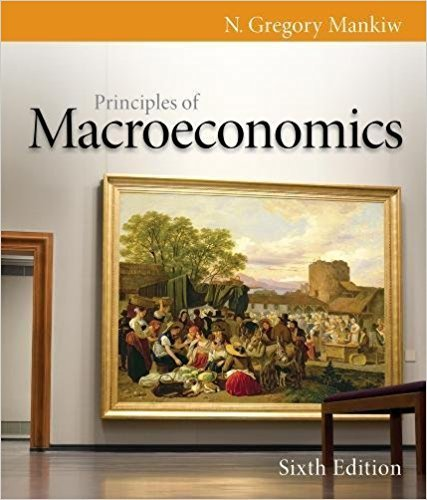×

×

# Solutions for Chapter 3: Interdependence and the Gains from Trade## Full solutions for Principles of Macroeconomics | 6th Edition

ISBN: 9780538453066Solutions for Chapter 3: Interdependence and the Gains from Trade

Solutions for Chapter 3
4 5 0 374 Reviews
18
2
##### ISBN: 9780538453066

Since 11 problems in chapter 3: Interdependence and the Gains from Trade have been answered, more than 9409 students have viewed full step-by-step solutions from this chapter. Chapter 3: Interdependence and the Gains from Trade includes 11 full step-by-step solutions. Principles of Macroeconomics was written by and is associated to the ISBN: 9780538453066. This expansive textbook survival guide covers the following chapters and their solutions. This textbook survival guide was created for the textbook: Principles of Macroeconomics, edition: 6.

Key Business Terms and definitions covered in this textbook
• average tax rate

total taxes paid divided by total income

• cost–benefit analysis

a study that compares the costs and benefits to society of providing a public good

• efficiency wages

above-equilibrium wages paid by firms to increase worker productivity

• efficient scale

the quantity of output that minimizes average total cost

• equilibrium

a situation in which the market price has reached the level at which quantity supplied equals quantity demanded

• marginal product

the increase in output that arises from an additional unit of input

• market

a group of buyers and sellers of a particular good or service

• natural monopoly

a monopoly that arises because a single firm can supply a good or service to an entire market at a smaller cost than could two or more firms

• negative income tax

a tax system that collects revenue from high-income households and gives subsidies to lowincome households

• normative statements

claims that attempt to prescribe how the world should be

• perfect substitutes

two goods with straight-line indifference curves

• permanent income

a person’s normal income

• present value

the amount of money today that would be needed, using prevailing interest rates, to produce a given future amount of money

• price elasticity of supply

a measure of how much the quantity supplied of a good responds to a change in the price of that good, computed as the percentage change in quantity supplied divided by the percentage change in price

• production function

the relationship between quantity of inputs used to make a good and the quantity of output of that good

• progressive tax

a tax for which highincome taxpayers pay a larger fraction of their income than do low-income taxpayers

• surplus

a situation in which quantity supplied is greater than quantity demanded

• transaction costs

the costs that parties incur in the process of agreeing to and following through on a bargain

• value of the marginal product

the marginal product of an input times the price of the output

• willingness to pay

the maximum amount that a buyer will pay for a good